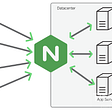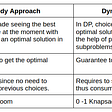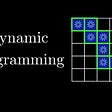# Blabla before diving into the question

Less is more. One of the very simple, however, very elegant idea in CS is two pointers. We can tread two layer for loop as two pointer problem. We can think the binary search as three pointers. The in place quick sort and quick select is using the two pointers. For this problem, 2 steps of the 3 key steps are using the idea of two pointers.

# Basic idea

We can divide the problems into 3 steps:

1. Reverse the whole string
2. Reverse each word
3. Remove extra space

For step 2 and step 3, we can use the two pointers to solve the problem.

For step two,

• if we have a space, we reset left and right to next location.
• If not a space, we increase right pointer

For step three, we have a similar problem 283. Move Zeroes. The solution is here:

`class Solution {public:    void moveZeroes(vector<int>& nums) {        int i = 0;        for (int j = 0; j < nums.size(); ++j)            if (nums[j])swap(nums[i++], nums[j]);    }};`

We can borrow the similar idea used for the third step.

# Reference Code

Based on all the above, we have the following solution in C++

`class Solution {public:    string reverseWords(string s) {        reverse(s.begin(), s.end());        s.push_back(' ');        // reverse each word        int left = 0, right = 0;        for (int i = 0; i < s.size(); ++i){            if (s[i] == ' '){                reverse(s.begin() + left, s.begin() + right);                left = i + 1; right = i + 1;            } else {                right++;            }        }        // remove the extra space        left = 0;        for (int i = 0; i < s.size(); ++i){            if (s[i] != ' '){                swap(s[i], s[left++]);                if (s[i+1] == ' ')left++;            }         }        s.erase(s.begin() + left - 1, s.end());        return s;    }};`

More my articles for different categories can be found in my personal website. Thanks for reading.

# Reference

--

--

--

## More from Jimmy Shen

Data Scientist/MLE/SWE @takemobi

Love podcasts or audiobooks? Learn on the go with our new app.

## Use NGINX hosting static websites## Model Design and Selection with Scikit-learn## NEW A.I. SOFTWARE | Instantly Transform Any Text Into A 100% Human-Sounding VoiceOver with only 3…## A Team Should Build the Most Impactful Parts of Its Software## Run Codenvy Anywhere## ADD.XYZ’s Weekly Update 26–Sprints are On!## Managed File Transfer (MFT) — Use Cases Series — Episode 1 — Salary File Transfer## Jimmy Shen

Data Scientist/MLE/SWE @takemobi

## Greedy Algorithms## Stacking Legoblocks into Stairs -Dynamic programming## Meeting Room Problems